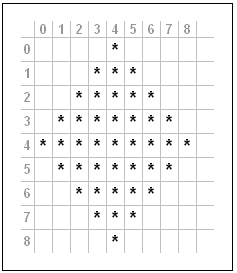### SODEVE

browse by category or date

# How To Draw Diamond Recursively

I had this interesting challenge from my colleague. He asked me to draw diamond recursively (not really draw, but plot using command line program :D). I managed to draw the 3×3 diamond, but I didn’t satisfied with my program. So I put more thought on it, and make it more interesting by allow us to define the width and the height of the diamond.```namespace ConsolePrograms
{
class DrawDiamond
{
static void Main(string[] args)
{
Console.WriteLine("1 Diamond, Width=7, Height=5");
SetupDiamond(1, 7, 5);
Console.WriteLine("4 Diamond, Width=3, Height=5");
SetupDiamond(4, 3, 5);
Console.WriteLine("9 Diamond, Width=3, Height=3");
SetupDiamond(9, 3, 3);
Console.WriteLine("16 Diamond, Width=7, Height=7");
SetupDiamond(16, 7, 7);
}

static void SetupDiamond(int NumberOfDiamonds, int Width, int Height)
{
//NumberOfDiamonds must be a Perfect Square
if (((int)Math.Pow(Math.Sqrt(NumberOfDiamonds),2.0)) != NumberOfDiamonds)
return;

//Width and Height must be an odd number bigger than 1
if (Width % 2 != 1 || Height % 2 != 1)
return;

int actualWidth = (int)Math.Sqrt(NumberOfDiamonds) * Width
- ((int)Math.Sqrt(NumberOfDiamonds)-1) ;

int actualHeight = (int)Math.Sqrt(NumberOfDiamonds) * Height
- ((int)Math.Sqrt(NumberOfDiamonds)-1);

int midWidth = (actualWidth - (actualWidth % 2)) / 2;
int midHeight = (actualHeight - (actualHeight % 2)) / 2;

Char[][] arCharacters = new Char[actualWidth ][];
for (int i = 0; i < actualWidth; i++)
arCharacters[i] = new Char[actualHeight];

for (int i = 0; i < actualWidth; i++)
for (int j = 0; j < actualHeight; j++)
arCharacters[i][j] = '.';

DrawDiamond(midWidth, 0, (Width-1)/2,
(Height-1)/2, midWidth, midHeight,
actualWidth, actualHeight,
ref arCharacters);

for (int i = 0; i < actualHeight ; i++)
{
for (int j = 0; j < actualWidth; j++)
Console.Write(arCharacters[j][i]);
Console.WriteLine();
}
}

public static void DrawDiamond(int x, int y, int Width,
int Height, int midWidth, int midHeight,
int actualWidth, int actualHeight,
ref Char[][] arCharacters)
{
if (x < 0 || x >= actualWidth)
return;

if (y >= actualHeight)
return;

arCharacters[x][y] = '*';

if (x < midWidth && y < midHeight)
{
DrawDiamond(x - Width, y + Height, Width,
Height, midWidth, midHeight,
actualWidth, actualHeight,
ref arCharacters);
}
else if (x > midWidth && y < midHeight)
{
DrawDiamond(x + Width, y + Height, Width,
Height, midWidth, midHeight,
actualWidth, actualHeight,
ref arCharacters);
}
else if (x < midWidth && y > midHeight)
{
DrawDiamond(x + Width, y + Height, Width,
Height, midWidth, midHeight,
actualWidth, actualHeight,
ref arCharacters);
}
else if (x > midWidth && y > midHeight)
{
DrawDiamond(x - Width, y + Height, Width,
Height, midWidth, midHeight,
actualWidth, actualHeight,
ref arCharacters);
}
else if (x == midWidth)
{
DrawDiamond(x - Width, y + Height, Width,
Height, midWidth, midHeight,
actualWidth, actualHeight,
ref arCharacters);
DrawDiamond(x + Width, y + Height, Width,
Height, midWidth, midHeight,
actualWidth, actualHeight,
ref arCharacters);
DrawDiamond(x, y + (Height*2), Width,
Height, midWidth, midHeight,
actualWidth, actualHeight,
ref arCharacters);
}
else if (x < midWidth && y == midHeight)
{
DrawDiamond(x + Width, y + Height, Width,
Height, midWidth, midHeight,
actualWidth, actualHeight,
ref arCharacters);
}
else if (x > midWidth && y == midHeight)
DrawDiamond(x - Width, y + Height, Width,
Height, midWidth, midHeight,
actualWidth, actualHeight,
ref arCharacters);
return;
}
}
}
```
GD Star Rating